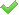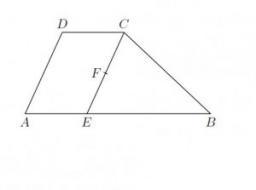# Trapezoid thirds

The ABCD trapezoid with the parallel sides of the AB and the CD and the E point of the AB side. The segment DE divides the trapezoid into two parts with the same area. Find the length of the AE line segment.

Result

x = (Correct answer is: )### Step-by-step explanation:Did you find an error or inaccuracy? Feel free to write us. Thank you!

Showing 1 comment:Dr Math
AE = 2/3 ABTips to related online calculators
Need help to calculate sum, simplify or multiply fractions? Try our fraction calculator.
Do you want to convert length units?

#### You need to know the following knowledge to solve this word math problem:

We encourage you to watch this tutorial video on this math problem:

## Related math problems and questions:

• Trapezoid MO-5-Z8ABCD is a trapezoid that lime segment CE is divided into a triangle and parallelogram, as shown. Point F is the midpoint of CE, DF line passes through the center of the segment BE, and the area of the triangle CDE is 3 cm2. Determine the area of the trape
• ABCD squareIn the ABCD square, the X point lies on the diagonal AC. The length of the XC is three times the length of the AX segment. Point S is the center of the AB side. The length of the AB side is 1 cm. What is the length of the XS segment?
• Diagonals at right angleIn the trapezoid ABCD, this is given: AB=12cm CD=4cm And diagonals crossed under a right angle. What is the area of this trapezoid ABCD?
• MO Z8–I–6 2018In the KLMN trapeze, KL has a 40 cm base and an MN of 16 cm. Point P lies on the KL line so that the NP segment divides the trapezoid into two parts with the same area. Find the length of the KP line.
• Rectangular trapezoidThe rectangular trapezoid ABCD is: /AB/ = /BC/ = /AC/. The length of the median is 6 cm. Calculate the circumference and area of a trapezoid.
• KLMN trapezoidThe KLMN trapezoid has bases KL 40cm and MN 16cm. On the KL base is point P. The segment NP divides the trapezoid into units with the same area. What is the distance of point P from point K?
• Diagonal intersectisosceles trapezoid ABCD with length bases | AB | = 6 cm, CD | = 4 cm is divided into 4 triangles by the diagonals intersecting at point S. How much of the area of the trapezoid are ABS and CDS triangles?
• MO Z9–I–2 - 2017In the VODY trapezoid, VO is a longer base and the diagonal intersection K divides the VD line in a 3:2 ratio. The area of the KOV triangle is 13.5 cm2. Find the area of the entire trapezoid.
• MO - trianglesOn the AB and AC sides of the triangle ABC lies successive points E and F, on segment EF lie point D. The EF and BC lines are parallel and is true this ratio FD:DE = AE:EB = 2:1. The area of ABC triangle is 27 hectares and line segments EF, AD, and DB seg
• Trapezoid - intersection of diagonalsIn the ABCD trapezoid is AB = 8 cm long, trapezium height 6 cm, and distance of diagonals intersection from AB is 4 cm. Calculate the trapezoid area.
• Trapezoid ABCD v2Trapezoid ABCD has a length of bases in ratio 3:10. The area of triangle ACD is 825 dm2. What is the area of trapezoid ABCD?
• A trapezoid 3A trapezoid ABCD has the bases length of a = 120 mm, c = 86 mm and the area A = 2,575 mm2. Find the height of the trapezoid.
• Trapezoid MOThe rectangular trapezoid ABCD with the right angle at point B, |AC| = 12, |CD| = 8, diagonals are perpendicular to each other. Calculate the perimeter and area of ​​the trapezoid.
• Trapezoid ABCDABDC is a trapezoid in which AB and CD are parallel sides measuring 6 and 9, respectively. Angles ABC and BCD are both right angles. Find the length of segment BD.
• Diagonal BDFind the length of the diagonal BD in a rectangular trapezoid ABCD with a right angle at vertex A when/AD / = 8,1 cm and the angle DBA is 42°
• Equilateral triangle ABCIn the equilateral triangle ABC, K is the center of the AB side, the L point lies on one-third of the BC side near the point C, and the point M lies in the one-third of the side of the AC side closer to the point A. Find what part of the ABC triangle cont
• Inner anglesThe magnitude of the internal angle at the main vertex C of the isosceles triangle ABC is 72°. The line p, parallel to the base of this triangle, divides the triangle into a trapezoid and a smaller triangle. How big are the inner angles of the trapezoid?